• 我想知道，模糊聚类分析第二步，应用相关系数法建立模糊相似矩阵的方法，用matlab怎么做？跪求，帮帮孩子吧，孩子不懂。</p>matlab 有问必答
• 模糊相似矩阵Matlab程序，%三次样条插值程序
• 模糊相似矩阵到模糊等价矩阵，通常采用的是传递闭包法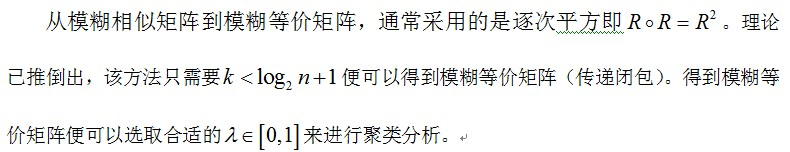模糊矩阵的乘法运算不同于普通的矩阵相乘，实质为取大取小运算。详细内容请查阅模糊数学相关书籍。
附上矩阵乘法代码：

Mat VagueMul(Mat& Q, Mat&R)
{
//Mat Q = (Mat_<float>(4,3)<< 0.3, 0.7, 0.2,   1, 0, 0.4,  0, 0.5, 1,    0.6, 0.7, 0.8 );
//Mat R = (Mat_<float>(3,2)<< 0.1, 0.9, 0.9, 0.1, 0.6, 0.4);
Mat VagueMat(Q.rows, R.cols, CV_32FC1);
vector<float> tmpFloatVector;
for (int i = 0; i < Q.rows; i++)
{
float* QData = Q.ptr<float>(i);
float* VagueData = VagueMat.ptr<float>(i);
for (int j = 0; j < R.cols; j++)
{
for (int k = 0; k < Q.cols; k++ )
{
tmpFloatVector.push_back(min(QData[k], R.ptr<float>(k)[j]));
}
VagueData[j] = *max_element(tmpFloatVector.begin(), tmpFloatVector.end());
tmpFloatVector.clear();
}
}
return VagueMat;
}

上述代码并不简洁，实际中的模糊相似矩阵往往为方阵，每次只需要计算上三角（或下三角），仅供参考。
转载请注明出处。
展开全文传递闭包
• 基于直觉模糊相似度和相异度，研究了直觉模糊相似矩阵构造问题。给出了直觉模糊相似度和相异度的标准定义，选取了有效的直觉模糊相似度量和相异度量方法；在分析了现有的几种直觉模糊相似矩阵构造方法基础上，提出了...
• 最优化及其matlab实现中模糊相似矩阵程序分析可以用到
• i求模糊相似矩阵的 MATLAB 程序 a=[276.2 324.5 158.6 412.5 292.8 258.4 334.1 303.2 292.9 243.2 159.7 331.2 251.5 287.3 349.5 297.4 227.8 453.6 321.5 451.0 466.2 307.5 421.1 455.1 192.7 433.2 289.9 366.3...
• 然而，现实生活中，事物之间的界限往往不一定很清晰，很多分类问题都伴随着模糊性。就拿黄色来说，深黄色、浅黄、米黄色之间就没有绝对界限，只能说此颜色跟某种颜色更相近，在计算机当中这个相近程度只能通过一定的...
在生产、科学实验以及日常生活中，常要求我们把所接触或者研究的领域，按照他们的性质用途等分成几类。然而，现实生活中，事物之间的界限往往不一定很清晰，很多分类问题都伴随着模糊性。就拿黄色来说，深黄色、浅黄、米黄色之间就没有绝对界限，只能说此颜色跟某种颜色更相近，在计算机当中这个相近程度只能通过一定的数学方法来表达。而用模糊数学的语言和方法对这类问题能够很好的解决，这就产生了模糊聚类分析。模糊聚类分析一般一般分为数据标准化、标定和聚类三步。
一、 模糊聚类分析：
1. 数据标准化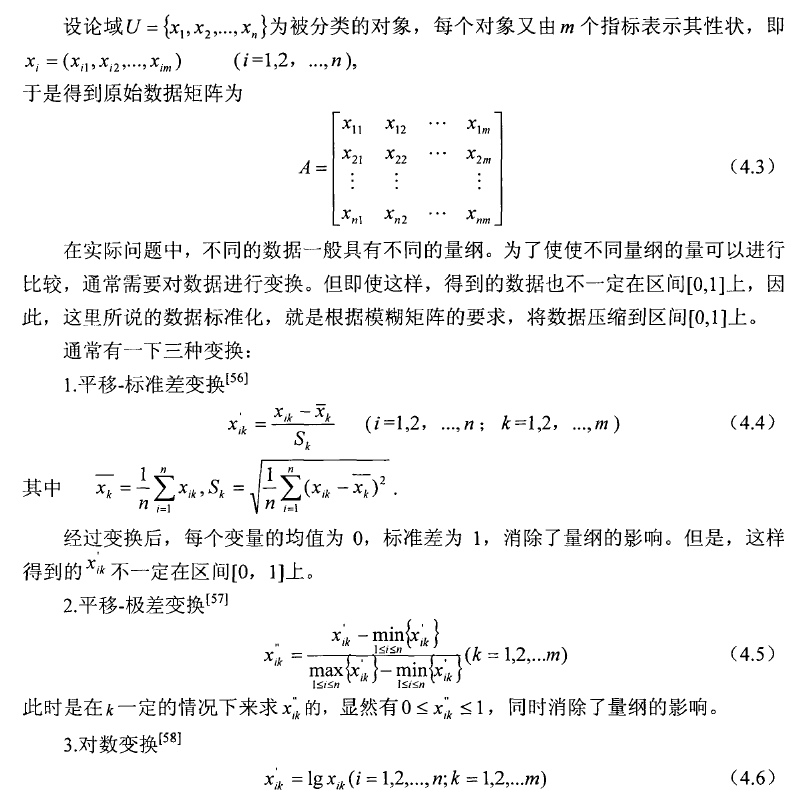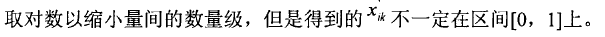2. 标定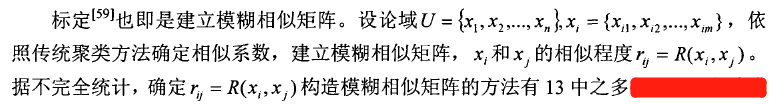根据需要，只介绍5种标定方法。 3. 聚类 因我只研究目标色与哪个颜色相近即可：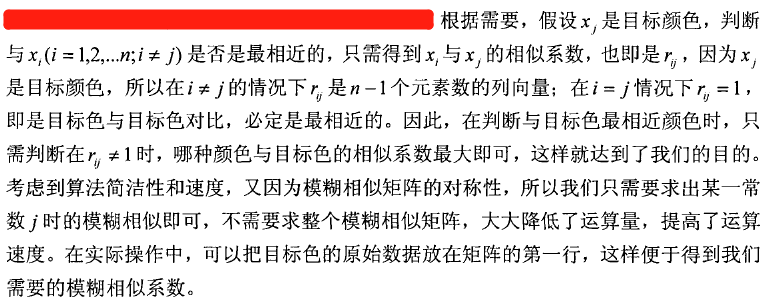二、 模糊相似矩阵的构造方法：
模糊相似矩阵的构造方法有13种之多，因篇幅有限，只对以下5种做简单介绍：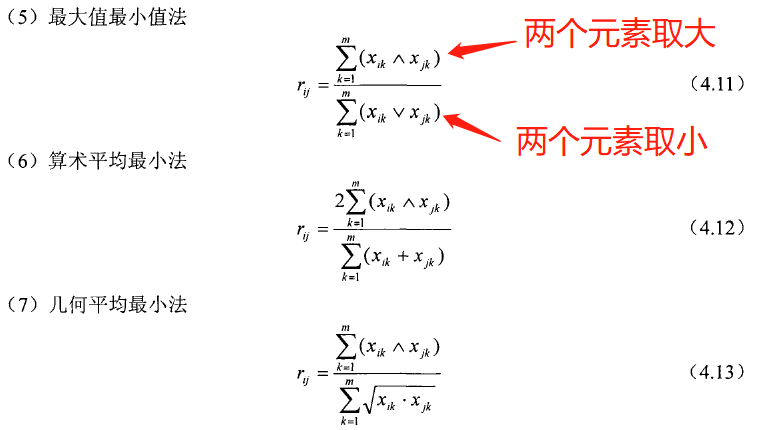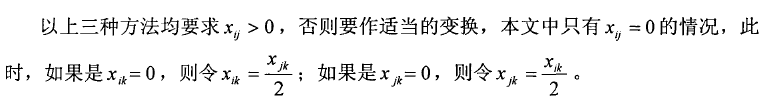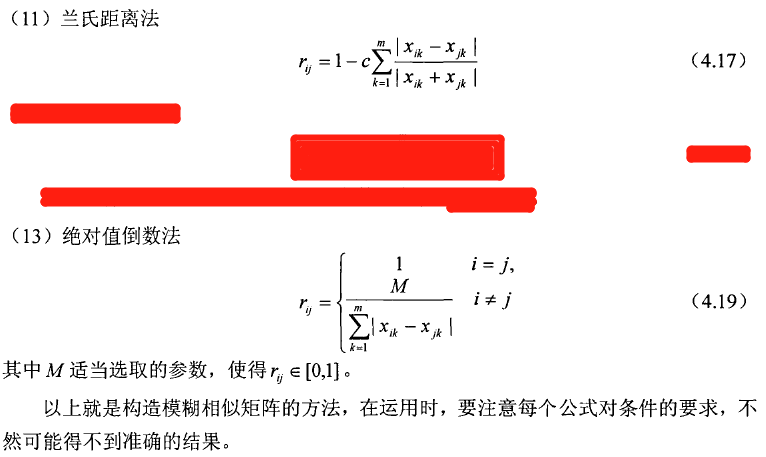三、 算法示例：
算法实现见下一篇博客。
如果有不明白的地方，参考知网论文：《基于模糊相似矩阵的尿糖试纸颜色识别研究》作者：谢彦斌
展开全文• //模糊相似矩阵实现 }; cpp文件： #include "FuzzySimilarMatrix.h" #include /**********均值************/ cv::Mat FSMatrix::Avg(cv::Mat Fuzzy) { cv::Mat A = Fuzzy; double sum = 0; cv::Mat Avg...
(VS2013+Opencv3.0) 本篇结合自己的研究项目，仅对算术平均最小值法、兰氏距离法（系数 c=0.1 时）、绝对值倒数法（系数 M=0.1 时和最大值_最小值法共4中相似矩阵的构造进行算法实现：
h文件：
#include<opencv2/opencv.hpp>
using namespace cv;
using namespace std;

class FSMatrix
{
public:
cv::Mat Avg(cv::Mat Fuzzy);//均值
cv::Mat sqrtD(cv::Mat sqrtd);//开根号
cv::Mat StandardDeviation(cv::Mat Standard);//标准差
cv::Mat Max_Min(cv::Mat Maxmin);//最大值_最小值法
cv::Mat Translation_standarddviation(cv::Mat Translation);//平移_标准差变换
cv::Mat Translation_range(cv::Mat Translation);//平移_极差变换
cv::Mat ArithmeticAverage_Min(cv::Mat AverageMin);//算术平均最小值法
cv::Mat Lance_distance(cv::Mat Lancedistance, double Lance_c);//兰氏距离法
cv::Mat AbsoluteValue_Reciprocal(cv::Mat AbsoluteReciprocal, double Reciprocal_c);//绝对值倒数法
cv::Mat FuzzySimilarMatrix(cv::Mat HSV, int i, double H, double S, double V);//模糊相似矩阵实现
};

cpp文件：
#include "FuzzySimilarMatrix.h"
#include<fstream>

/**********均值************/
cv::Mat FSMatrix::Avg(cv::Mat Fuzzy)
{
cv::Mat A = Fuzzy;
double sum = 0;
cv::Mat Avg(1, A.cols, CV_64FC1);
cv::Mat s(1, A.cols, CV_64FC1);
for (int j = 0; j < A.cols; j++)  //列
{
sum = A.at<double>(0, j);
for (int i = 1; i < A.rows; i++)  //行
{

sum += A.at<double>(i, j);
}
Avg.at<double>(0, j) = sum / A.rows;
}
//cout << "Avg =" << Avg << endl;
return  Avg;
}

/**********开根号************/
cv::Mat FSMatrix::sqrtD(cv::Mat sqrtd)
{
cv::Mat sq(sqrtd.rows, sqrtd.cols, CV_64FC1);
for (int i = 0; i<sqrtd.rows; i++)
{
for (int j = 0; j<sqrtd.cols; j++)  //列
{
sq.at<double>(i, j) = sqrt(sqrtd.at<double>(i, j));

double xxx = sq.at<double>(i, j);
}
}
//cout << "sq =" << sq << endl;
return sq;
}

/**********标准差************/
cv::Mat FSMatrix::StandardDeviation(cv::Mat Standard)
{
cv::Mat s(Standard.rows, Standard.cols, CV_64FC1);
cv::Mat C(1, Standard.cols, CV_64FC1);
C = Avg(Standard);
double sum = 0;
if (s.cols)
{
for (int j = 0; j<Standard.cols; j++)    //列
{
for (int i = 0; i<Standard.rows; i++)
{
s.at<double>(i, j) = Standard.at<double>(i, j) - C.at<double>(0, j);
//s.at<double>(i, j) = (s.at<double>(i, j) * s.at<double>(i, j));
s.at<double>(i, j) = (s.at<double>(i, j) * s.at<double>(i, j));
//	cout << "S =" << s.at<double>(i, j) << endl;
}

}

C = Avg(s);
//	cout << "C_Avg =" << C << endl;
C = sqrtD(C);
}
//	cout << "C =" << C << endl;
return C;
}

/*********平移_标准差变换**********/
cv::Mat  FSMatrix::Translation_standarddviation(cv::Mat Translation)
{
cv::Mat S(1, Translation.cols, CV_64FC1);
cv::Mat Av(1, Translation.cols, CV_64FC1);
cv::Mat T(Translation.rows, Translation.cols, CV_64FC1);

S = StandardDeviation(Translation);
Av = Avg(Translation);
for (int j = 0; j<Translation.cols; j++)    //列
{
for (int i = 0; i<Translation.rows; i++)
{
T.at<double>(i, j) = (Translation.at<double>(i, j) - Av.at<double>(0, j)) / S.at<double>(0, j);
}
}
cout << "T =" << T << endl;
return T;
}

/*********平移_极差变换********/
cv::Mat FSMatrix::Translation_range(cv::Mat Translation)
{
cv::Mat Range = Translation_standarddviation(Translation);
cv::Mat Range_max = cv::Mat::zeros(cv::Size(Range.cols, 1), CV_64FC1);
cv::Mat Range_min = cv::Mat::zeros(cv::Size(Range.cols, 1), CV_64FC1);
for (int j = 0; j<Range.cols; j++)    //列
{
Range_max.at<double>(0, j) = Range.at<double>(0, j);
Range_min.at<double>(0, j) = Range.at<double>(0, j);
for (int i = 1; i< Range.rows; i++)
{
if (Range_max.at<double>(0, j) < Range.at<double>(i, j))
{
Range_max.at<double>(0, j) = Range.at<double>(i, j);
//cout << "Range_max =" << Range_max << endl;
}
if (Range_min.at<double>(0, j) > Range.at<double>(i, j))
{
Range_min.at<double>(0, j) = Range.at<double>(i, j);
//cout << "Range_min =" << Range_min << endl;
}
}
}

cout << "Range_max =" << Range_max << endl;
cout << "Range_min =" << Range_min << endl;

for (int j = 0; j<Range.cols; j++)    //列
{
for (int i = 0; i< Range.rows; i++)
{
Range.at<double>(i, j) = (Range.at<double>(i, j) - Range_min.at<double>(0, j)) / (Range_max.at<double>(0, j) - Range_min.at<double>(0, j));
}
}
cout << "Range =" << Range << endl;
return Range;
}

/*********矩阵内元素为0时，调整********/
{
for (int i = 1; i < Adjustment.rows; i++)    //行
{
for (int j = 0; j < Adjustment.cols; j++)
{
{
}
}
}
}

/*********最大值_最小值法********/
cv::Mat FSMatrix::Max_Min(cv::Mat Maxmin)
{
cv::Mat R(Maxmin.rows, 1, CV_64FC1);
//cout << "Maxmin =" << Maxmin << endl;
for (int i = 0; i<Maxmin.rows; i++)    //行
{
double SumMax = 0;
double SumMin = 0;
double SumMaxMin = 0;
for (int j = 0; j < Maxmin.cols; j++)
{
double Max = Maxmin.at<double>(0, j);
double Min = Maxmin.at<double>(0, j);

if (Max <= Maxmin.at<double>(i, j))
{
Max = Maxmin.at<double>(i, j);
}
SumMax += Max;
if (Min >= Maxmin.at<double>(i, j))
{
Min = Maxmin.at<double>(i, j);
}
SumMin += Min;

}
SumMaxMin = SumMin / SumMax;

R.at<double>(i, 0) = SumMin / SumMax;

ofstream oFile;
oFile.open("Max_Min.csv", ios::out | ios::trunc);
oFile << R << endl;
oFile.close();

}
return R;
}

/*********算术平均最小值法********/
cv::Mat FSMatrix::ArithmeticAverage_Min(cv::Mat AverageMin)
{
cv::Mat A(AverageMin.rows, 1, CV_64FC1);
//cout << "AverageMin =" << AverageMin << endl;
for (int i = 0; i<AverageMin.rows; i++)    //行
{
double SumMin = 0;
double ArithmeticAverageMin = 0;
double S = 0;
for (int j = 0; j < AverageMin.cols; j++)
{
S += AverageMin.at<double>(0, j);
double Min = AverageMin.at<double>(0, j);

if (Min >= AverageMin.at<double>(i, j))
{
Min = AverageMin.at<double>(i, j);
}
SumMin += Min;
S += AverageMin.at<double>(i, j);
}

ArithmeticAverageMin = (2*SumMin) / S;
//cout << "ArithmeticAverageMin =" << ArithmeticAverageMin << endl;
A.at<double>(i, 0) = (2 * SumMin) / S;

ofstream oFile;
oFile.open("ArithmeticAverage_Min.csv", ios::out | ios::trunc);
oFile << A << endl;
oFile.close();

}
return A;
}

/*********兰氏距离法********/
cv::Mat FSMatrix::Lance_distance(cv::Mat Lancedistance, double Lance_c)
{
cv::Mat L(Lancedistance.rows, 1, CV_64FC1);
//cout << "Lancedistance =" << Lancedistance << endl;
for (int i = 0; i<Lancedistance.rows; i++)    //行
{
double S1 = 0;
double S2 = 0;
double S = 0;
for (int j = 0; j < Lancedistance.cols; j++)
{
S1 = abs(Lancedistance.at<double>(0, j) - Lancedistance.at<double>(i, j));
S2 = abs(Lancedistance.at<double>(0, j) + Lancedistance.at<double>(i, j));
S += S1 / S2;
}
L.at<double>(i, 0) = 1 - (Lance_c * S) ;
ofstream oFile;
oFile.open("Lancedistance.csv", ios::out | ios::trunc);
oFile << L << endl;
oFile.close();
}
return L;
}

/*********绝对值倒数法********/
cv::Mat FSMatrix::AbsoluteValue_Reciprocal(cv::Mat AbsoluteReciprocal, double Reciprocal_c)
{
cv::Mat Ab(AbsoluteReciprocal.rows, 1, CV_64FC1);
//cout << "AbsoluteReciprocal =" << AbsoluteReciprocal << endl;
for (int i = 0; i<AbsoluteReciprocal.rows; i++)    //行
{
double S1 = 0;
for (int j = 0; j < AbsoluteReciprocal.cols; j++)
{
S1+= abs(AbsoluteReciprocal.at<double>(0, j) - AbsoluteReciprocal.at<double>(i, j));
}
Ab.at<double>(i, 0) = (Reciprocal_c / S1);
Ab.at<double>(0, 0) = 1;

ofstream oFile;
oFile.open("AbsoluteReciprocal.csv", ios::out | ios::trunc);
oFile << Ab << endl;
oFile.close();
}
return Ab;
}

/*********模糊相似矩阵实现********/
cv::Mat FSMatrix::FuzzySimilarMatrix(cv::Mat HSV, int i, double H, double S, double V)
{
int row = 0;//有几行，即几个参数
for (int j = 0; j<HSV.rows; j++)
{
if (HSV.at<double>(j, 0) == i)
{
row++;//有几行，即几个参数
cout << "HSV.at<double>(j, 0) =" << HSV.at<double>(j, 0) << endl;  //调用文档(HSV.txt)的几行,即HSV矩阵的几行
}
}

cv::Mat HSVR = cv::Mat::zeros(cv::Size(3, row+1), CV_64FC1);
if (row)
{
int j0 = 1;
HSVR.at<double>(0, 0) = H;
HSVR.at<double>(0, 1) = S;
HSVR.at<double>(0, 2) = V;
for (int j = 0; j < HSV.rows; j++)
{
if (HSV.at<double>(j, 0) == i)
{
HSVR.at<double>(j0, 0) = HSV.at<double>(j, 1);
HSVR.at<double>(j0, 1) = HSV.at<double>(j, 2);
HSVR.at<double>(j0, 2) = HSV.at<double>(j, 3);
j0++;
}
}
cout << "HSVR =" << HSVR << endl; //HSVR为原始数据矩阵，第一行为H、S、V值
}
cv::Mat Mm;
cv::Mat Tr;
cv::Mat AverageMin;
Tr = Translation_range(HSVR);
cout << "Max_Min =" << Mm << endl;

cout << "AverageMin =" << AverageMin << endl;

cv::Mat Lance;
Lance = Lance_distance(Tr, 0.1);
cout << "Lance =" << Lance << endl;

cv::Mat Rec;
Rec = AbsoluteValue_Reciprocal(Tr, 0.1);
cout << "Rec =" << Rec << endl;

ofstream oFilese2("Max_MinCSV.csv", ios::app);
oFilese2 << Mm << endl;
oFilese2.close();

return Mm;
}

main主函数调用：
#include<fstream>
#include "FuzzySimilarMatrix.h"
using namespace std;
using namespace cv;

void main()
{
ifstream file;//创建文件流对象
file.open("HSV.txt");
cv::Mat Hhsv = cv::Mat::zeros(64, 4, CV_64FC1);//创建Mat类矩阵，定义初始化值全部是0，矩阵大小和txt一致
//将txt文件数据写入到Hhsv矩阵中
for (int ii = 0; ii < 64; ii++)
{
for (int j = 0; j < 4; j++)
{
file >> Hhsv.at<double>(ii, j);
}
}
FSMatrix FSm;
FSm.FuzzySimilarMatrix(Hhsv, 10, 166, 67, 162);
}

运行结果展示：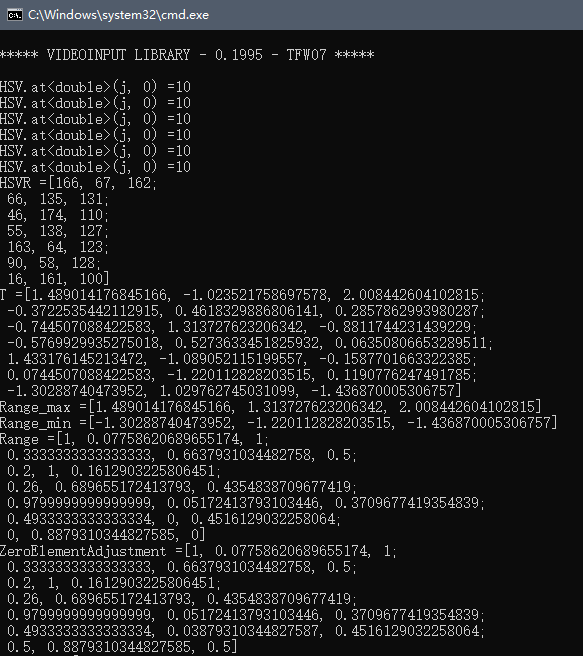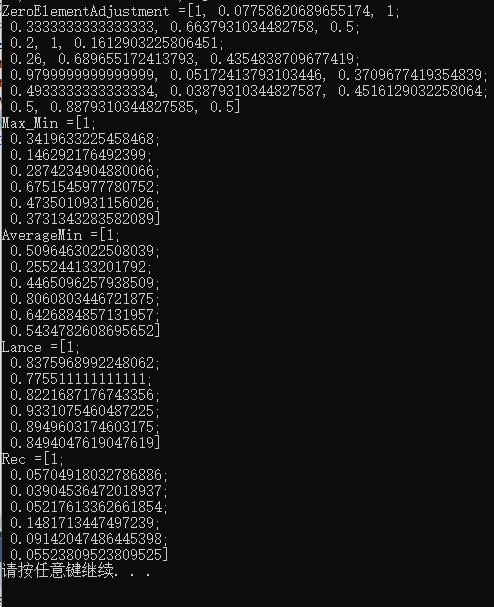注意：HSV.txt文档部分数据：（64行4列）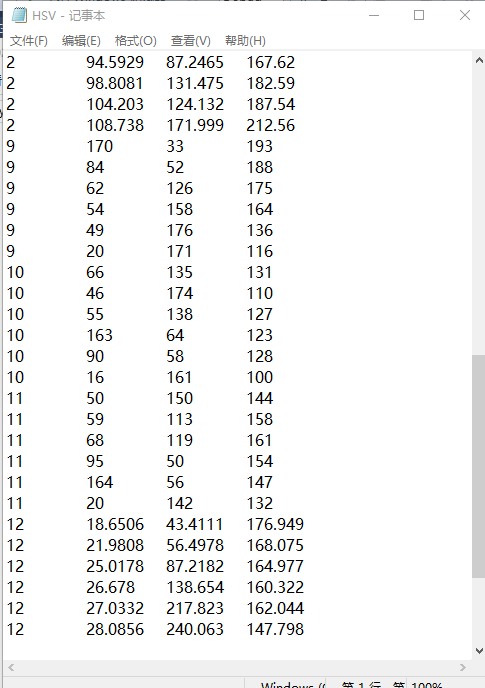展开全文opencv
• 模糊聚类中的标准化、模糊相似矩阵、模糊等价矩阵的建立。
• 给出一个模糊相似矩阵的实例,存在与该矩阵距离相同且都是最小的两个不相等的模糊等价矩阵,从而证明了全局最优模糊等价矩阵不具有唯一性.对基于摄动的模糊聚类算法求出的可行解的不同情况进行分析,给出了每种情况下...
• 给出一个模糊相似矩阵的实例,存在与该矩阵距离相同且都是最小的两个不相等的模糊等价矩阵,从而证明了全局最优模糊等价矩阵不具有唯一性.对基于摄动的模糊聚类算法求出的可行解的不同情况进行分析,给出了每种情况下...
• 模糊数学模型系列博文： ...【3】模糊聚类分析方法：模糊等价矩阵、模糊相似矩阵、传递闭包法、布尔矩阵法 【4】模糊决策分析方法 目录 1.1 模糊数学简介 1.2.1 模糊集和隶属函数 1.2.2 模糊集合的表示方法 ...
模糊数学模型系列博文：
【1】基本概念： 隶属函数、模糊集合的表示方法、模糊关系、模糊矩阵
【2】模糊模式识别：海明贴近度 、欧几里得贴近度 、黎曼贴近度、 格贴近度、最大隶属原则、择近原则
【3】模糊聚类分析方法：模糊等价矩阵、模糊相似矩阵、传递闭包法、布尔矩阵法
【4】模糊决策分析方法

目录
1.1 模糊数学简介
1.2.1 模糊集和隶属函数
1.2.2 模糊集合的表示方法
i) zadeh 表示法                          ii) 序偶表示法                            iii) 向量表示法
1.2.3 模糊集的运算
1.2.4 隶属函数的确定方法
（1）模糊统计方法                             （2）指派方法
1.3 模糊关系、模糊矩阵
1.3.1 基本概念
1.3.2 模糊矩阵的运算及其性质
(1) 模糊矩阵间的关系及并、交、余运算                               (2) 模糊矩阵的合成
(3) 模糊矩阵的转置                                                                 (4) 模糊矩阵的λ − 截矩阵

1.1 模糊数学简介
1965 年，美国著名计算机与控制专家查德(L.A.Zadeh)教授提出了模糊的概念，并 在国际期刊《Information and Control》并发表了第一篇用数学方法研究模糊现象的论文 “Fuzzy Sets”(模糊集合)，开创了模糊数学的新领域。 模糊是指客观事物差异的中间过渡中的“不分明性”或“亦此亦彼性”。如高个子 与矮个子、年轻人与老年人、热水与凉水、环境污染严重与不严重等。在决策中，也有 这种模糊的现象，如选举一个好干部，但怎样才算一个好干部？好干部与不好干部之间 没有绝对分明和固定不变的界限。这些现象很难用经典的数学来描述。 模糊数学就是用数学方法研究与处理模糊现象的数学。它作为一门崭新的学科，它 是继经典数学、统计数学之后发展起来的一个新的数学学科。经过短暂的沉默和争议之 后，迅猛的发展起来了，而且应用越来越广泛。如今的模糊数学的应用已经遍及理、工、 农、医及社会科学的各个领域，充分的表现了它强大的生命力和渗透力。 统计数学是将数学的应用范围从确定性的领域扩大到了不确定性的领域，即从必然 现象到偶然现象，而模糊数学则是把数学的应用范围从确定领域扩大到了模糊领域，即 从精确现象到模糊现象。 实际中，我们处理现实的数学模型可以分成三大类：第一类是确定性数学模型，即 模型的背景具有确定性，对象之间具有必然的关系。第二类是随机性的数学模型，即模 型的背景具有随机性和偶然性。第三类是模糊性模型，即模型的背景及关系具有模糊性。
1.2 基本概念
1.2.1 模糊集和隶属函数
【定义 1】 论域 X 到[0,1]闭区间上的任意映射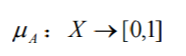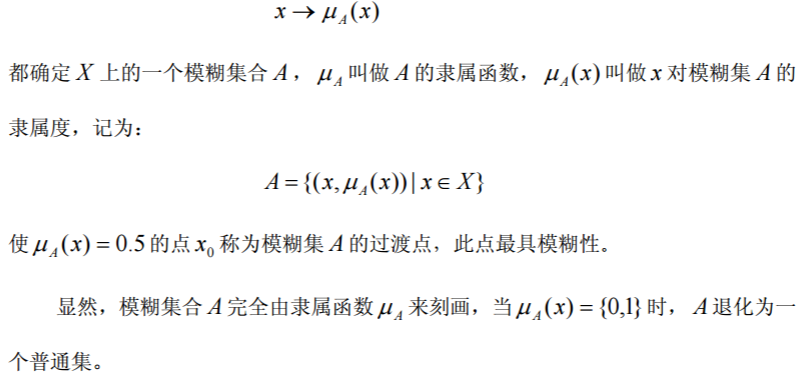1.2.2 模糊集合的表示方法
当论域 X 为有限集时，记 ，则 X 上的模糊集 A 有下列三种常 见的表示形式。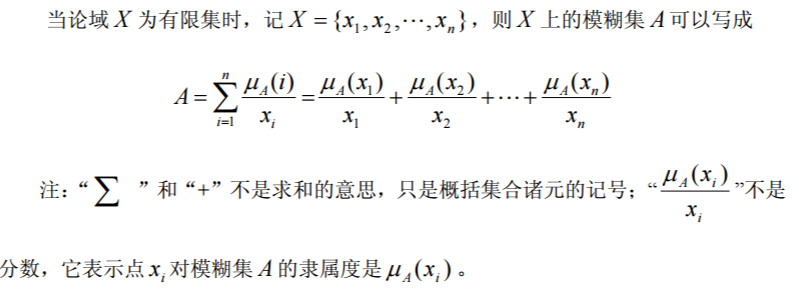ii) 序偶表示法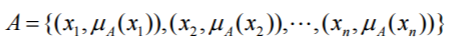iii) 向量表示法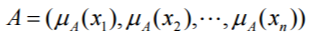当论域 X 为无限集时， X 上的模糊集 A 可以写成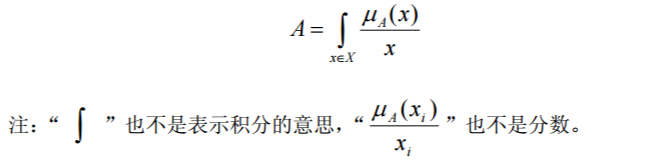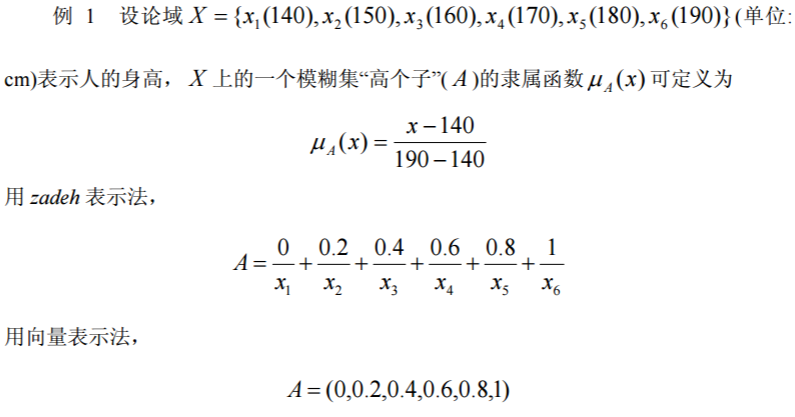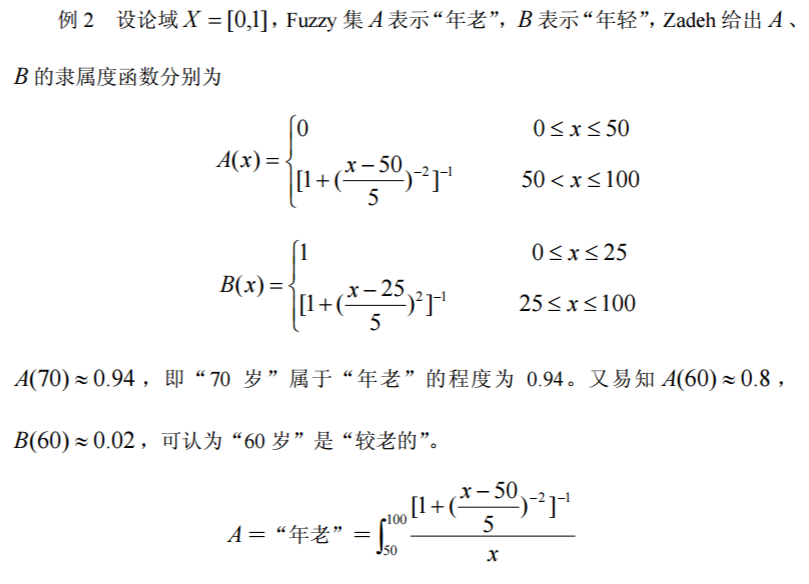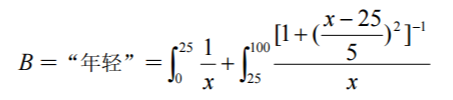1.2.3 模糊集的运算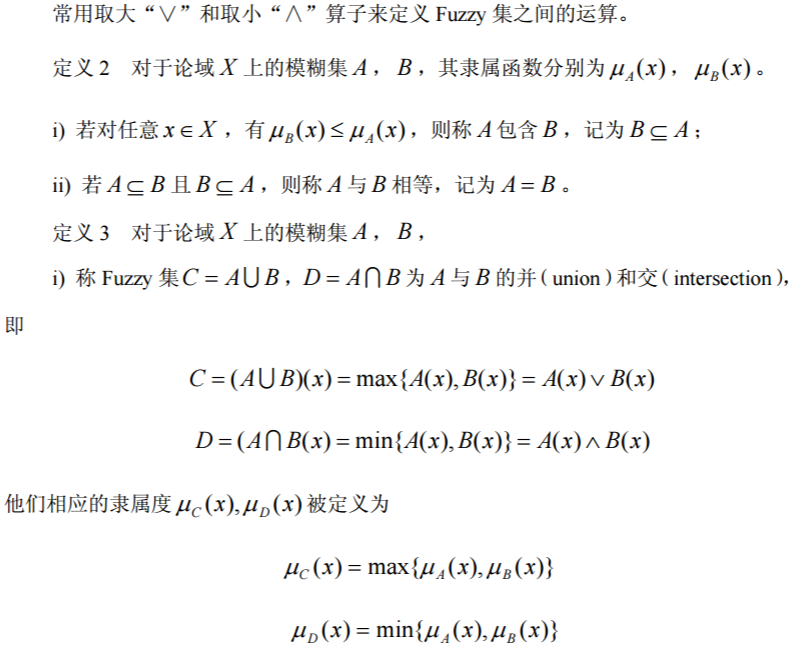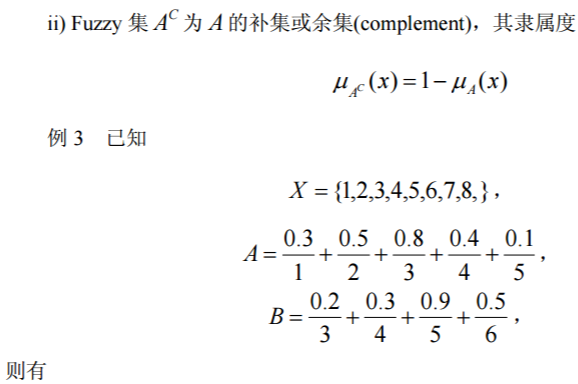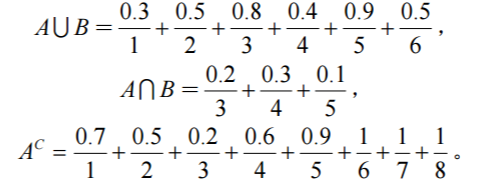1.2.4 隶属函数的确定方法
模糊数学的基本思想是隶属度的思想。应用模糊数学方法建立数学模型的关键是建 立符合实际的隶属函数。如何确定一个模糊集的隶属函数至今还是尚未解决的问题。这 里仅仅介绍几种常用的确定隶属函数的方法。
（1）模糊统计方法
模糊统计方法是一种客观方法，主要是基于模糊统计试验的基础上根据隶属度的客 观存在性来确定的。所谓的模糊统计试验包含以下四个要素：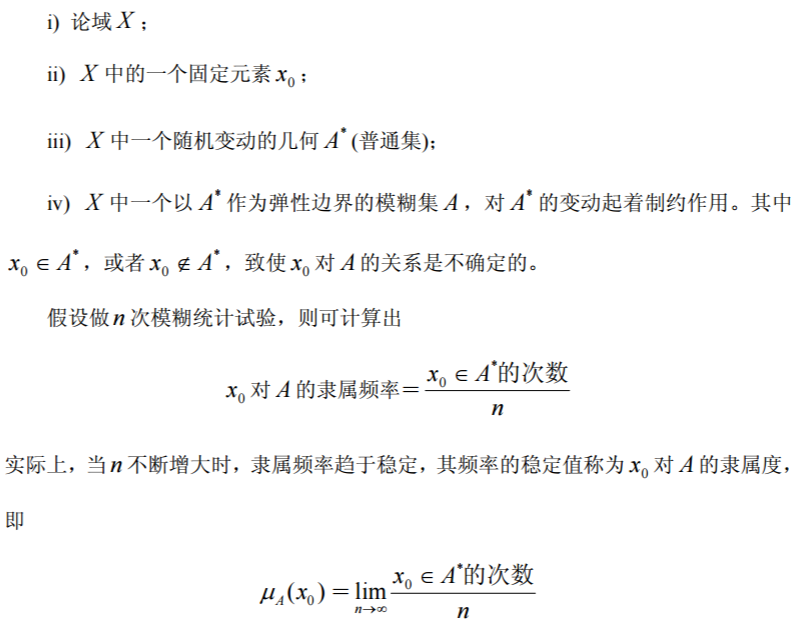（2）指派方法
指派方法是一种主观的方法，它主要依据人们的实践经验来确定某些模糊集隶属函 数的一种方法。
如果模糊集定义在实数域 R 上，则模糊集的隶属函数称为模糊分布。所谓指派方 法就是根据问题的性质主观地选用某些形式地模糊分布，再根据实际测量数据确定其中 所包含地参数，常用的模糊分布如表 1 所示。 实际中，根据问题对研究对象的描述来选择适当的模糊分布：
① 偏小型模糊分布一般适合于描述像“小，少，浅，淡，冷，疏，青年”等偏小 的程度的模糊现象。
② 偏大型模糊分布一般适合于描述像“大，多，深，浓，热，密，老年”等偏大 的程度的模糊现象。
③ 中间型模糊分布一般适合于描述像“中，适中，不太多，不太少，不太深，不 太浓，暖和，中年”等处于中间状态的模糊现象。 但是，表 1 给出的隶属函数都是近似的，应用时需要对实际问题进行分析，逐步修 改进行完善，最后得到近似程度更好的隶属函数。
（3）其它方法
在实际应用中，用来确定模糊集的隶属函数的方法示多种多样的，主要根据问题的 实际意义来确定。譬如，在经济管理、社会管理中，可以借助于已有的“客观尺度”作 为模糊集的隶属度。下面举例说明。
如果设论域 X 表示机器设备，在 X 上定义模糊集 A ＝“设备完好”，则可以用“设 备完好率”作为 A 的隶属度。如果 X 表示产品，在 X 上定义模糊集 A ＝“质量稳定”， 则可以用产品的“正品率”作为 A 的隶属度。如果 X 表示家庭，在 X 上定义模糊集 A ＝“家庭贫困”，则可以用“Engel 系数＝食品消费/总消费”作为 A 的隶属度。
另外，对于有些模糊集而言，直接给出隶属度有时是很困难的，但可以利用所谓的 “二元对比排序法”来确定，即首先通过两两比较确定两个元素相应隶属度的大小排出 顺序，然后用数学方法加工处理得到所需的隶属函数。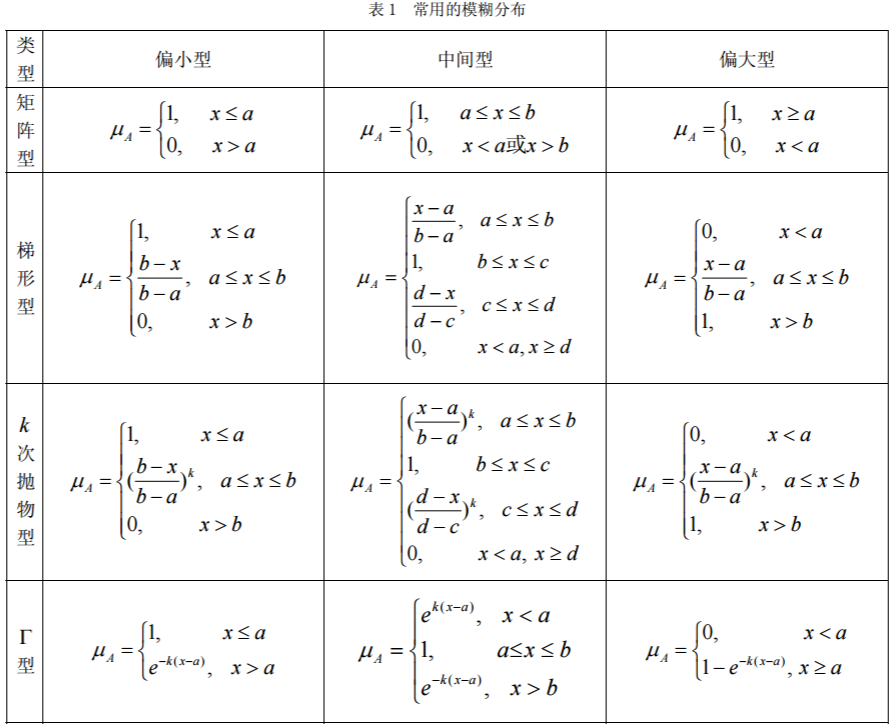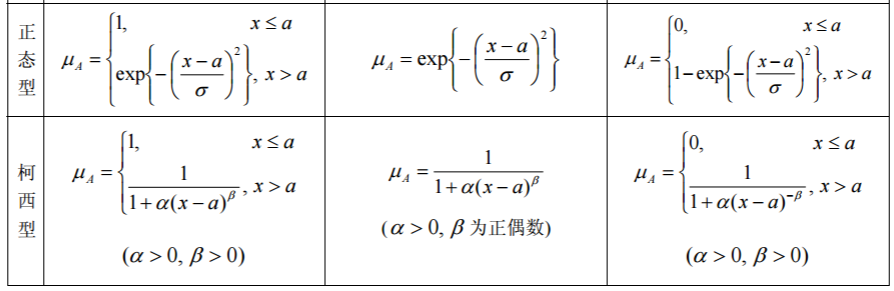1.3 模糊关系、模糊矩阵
1.3.1 基本概念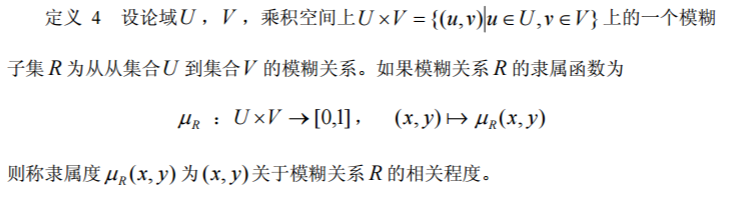这是二元模糊关系的数学定义，多元模糊关系也可以类似定义。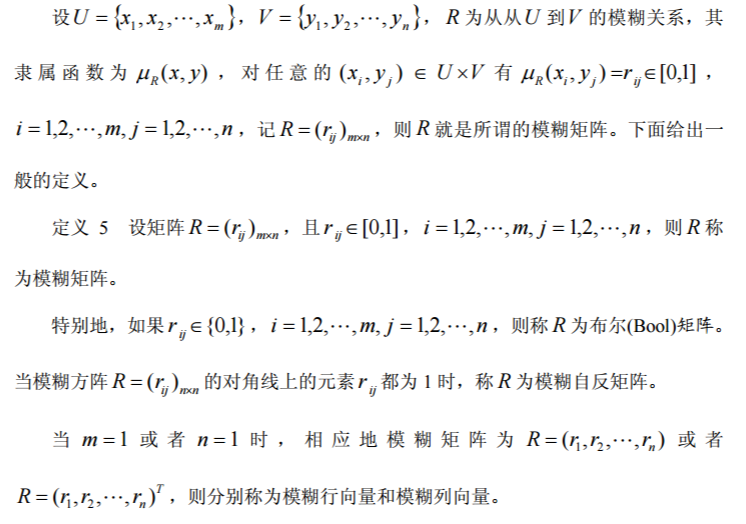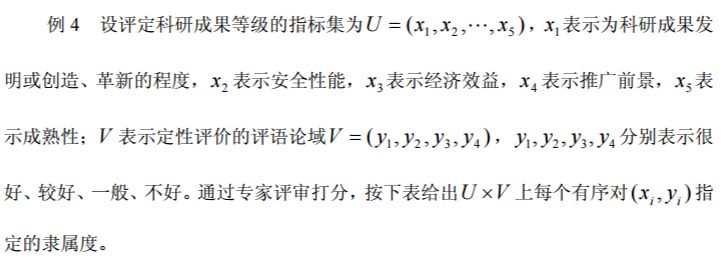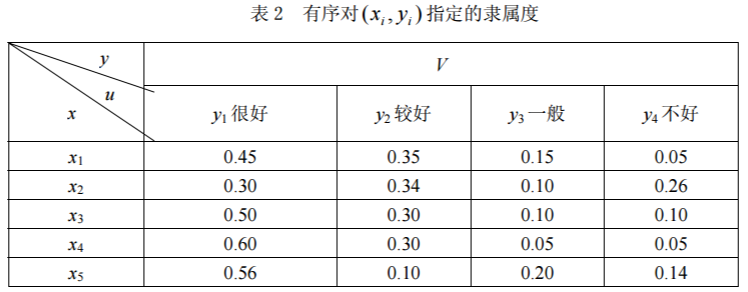由此确定一个从U 到V 的模糊关系 R ，这个模糊关系的隶属度函数是一个 5×4 阶 的矩阵，记为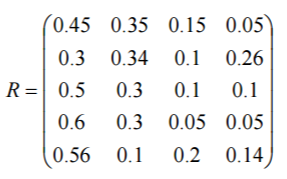则 R 为一个模糊关系矩阵。
1.3.2 模糊矩阵的运算及其性质
(1) 模糊矩阵间的关系及并、交、余运算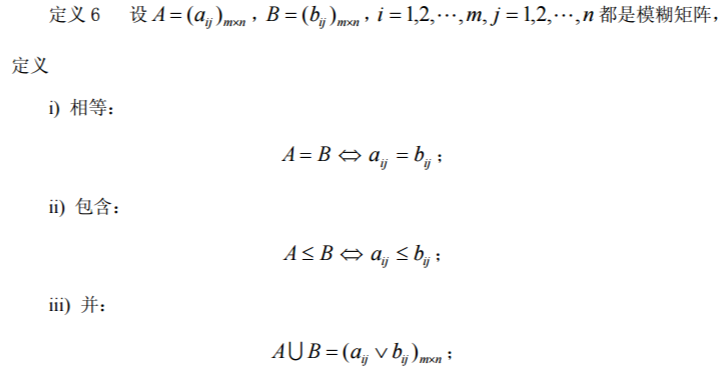(2) 模糊矩阵的合成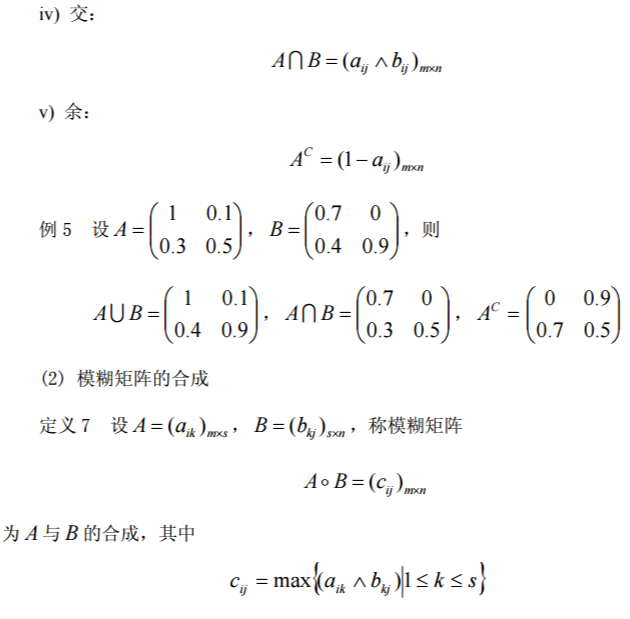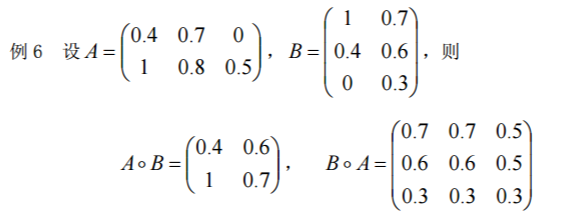两模糊矩阵合成的 MATLAB 函数如下：
function ab=synt(a,b);
m=size(a,1);n=size(b,2);
for i=1:m
for j=1:n
ab(i,j)=max(min([a(i,:);b(:,j)']));
end
end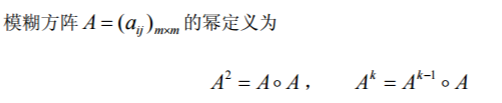(3) 模糊矩阵的转置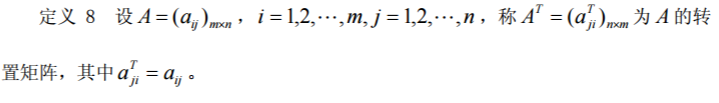(4) 模糊矩阵的λ − 截矩阵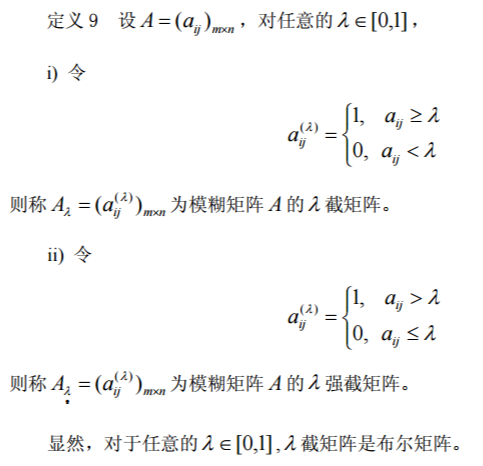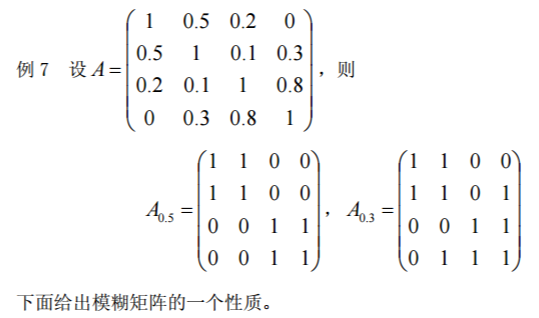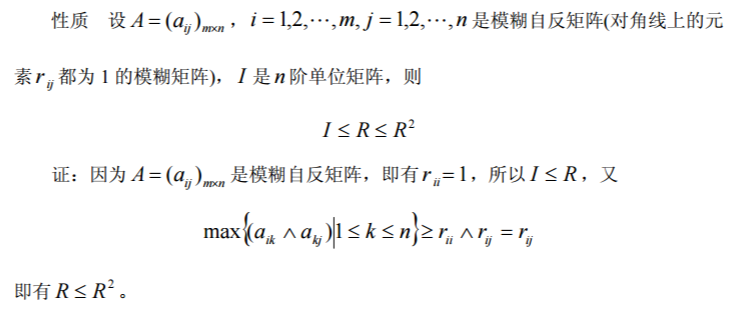模糊数学模型系列博文：
【1】基本概念： 隶属函数、模糊集合的表示方法、模糊关系、模糊矩阵
【2】模糊模式识别：海明贴近度 、欧几里得贴近度 、黎曼贴近度、 格贴近度、最大隶属原则、择近原则
【3】模糊聚类分析方法：模糊等价矩阵、模糊相似矩阵、传递闭包法、布尔矩阵法
【4】模糊决策分析方法


展开全文• 已知子女与父母相似关系的模糊矩阵R和父母与祖父母相似关系的模糊矩阵S分别如下所示，求子女与祖父母的相似关系模糊矩阵。word文档有具体描述。
• 求大神赐教：用C对大约300个数据进行模糊矩阵分析以及求其模糊相似矩阵c 数据
• 模糊相似关系方程Ｘ２＝Ｘ的分解构造理论基础上，用泛函分析方法证明了与给定的模糊相似阵距离最近的最优模糊等价阵的存在性与局部唯一性；还证明了局部最优模糊等价阵...
• 然后定义了直觉模糊相似矩阵的合成运算法则,给出直觉模糊相似矩阵转化为直觉模糊等价矩阵的途径;此外,还分别定义了直觉模糊相似矩阵和直觉模糊等价矩阵的λ-截矩阵,进而给出了直觉模糊集的一种聚类方法;最后通过算例...
• 采用作者发现的模糊相似矩阵传递闭包的Warshall算法进行模糊聚类,时间复杂度比平方法低。用C语言设计了模糊相似矩阵夹角余弦标定法、Warshall算法计算传递闭包、置信水平排序、动态聚类模块,用菜单驱动,并对算法进行...
• 针对现有构造方法缺少含义的问题，尤其是针对模糊问题，提出了一种基于最小信息熵值聚类构造相似矩阵的方法．该方法首先利用最小信息熵值原理获得多准则决策中不同属性的权重信息，然后求解不同方案集间的加权距离．...
• 用Matlab建立的模糊等价矩阵，十分有用，解决了不同阶次的矩阵求等价矩阵的问题，欢迎分享
• ## python计算相似矩阵

千次阅读 2018-06-08 00:10:24
模糊数学课上完之后对使用python中的sklearn计算矩阵各个元素的相似度有了一些感悟。 首先需要强调的是python中对于矩阵的计算尽量不要在DataFrame中，效率非常低。应该使用numpy。 1 预处理 常用1:...
• 从隶属度函数可以得到隶属度，隶属度是一个数，数可以组成向量与矩阵，叫模糊矩阵，我们介绍下模糊数学里面见得比较多的一些矩阵，看一下他们究竟是什么东西。         ...
•数学建模 评价模型
• 然后定义了直觉模糊相似矩阵的合成运算法则，给出直觉模糊相似矩阵转化为直觉模糊等价矩阵的途径；此外，还分别定义了直觉模糊相似矩阵和直觉模糊等价矩阵的λ-截矩阵，进而给出了直觉模糊集的一种聚类方法；最后...
• 一般来说，由不同方法构造的模糊关系矩阵只是一个模糊相似矩阵，不一定具有传递性。因此要从R出发构造出一个新的模糊等价矩阵，然后以此等价矩阵作为基础，进行动态聚类。模糊相似矩阵R的传递闭包t(R)就是一个模糊...
• 程序可以实现模糊聚类运算，包括求标准矩阵，模糊相似矩阵，模糊等价矩阵和截矩阵
• 通过Web日志中记录的客户对Web页面的访问情况建立Web页面的用户访问矩阵，在此基础上构造模糊相似矩阵，根据模糊相似矩阵由最大树算法进行聚类。分析和算例表明，通过模糊相似矩阵进行聚类避免了构造模糊等价矩阵的...数据挖掘 Web日志 Web使用挖掘 最大树法
• 分析了基于摄动的模糊聚类方法(fuzzy clustering method based on perturbation,FCMBP),指出指数复杂度的遍历过程是目前PC计算环境下难以处理十阶以上较高阶数模糊相似矩阵的原因.把寻求具有最小"失真"的最优模糊...
•  这篇文章估计是这个...本文主要讲的是相似矩阵的一些事情，本文的观点很是粗糙，自己感觉都有点模糊，因此请读者细细阅读。在孟岩的文章里头，它对矩阵及其相似有了一个非常精彩的描述： “矩阵是线性空间中
• 在区间量的相似性度量基础上，将属性集映射到论域笛卡尔积上的一个模糊集簇，建立了基于模糊区分矩阵的属性约简理论，讨论了其特点，提出了将区分度离散化的观点，探讨了它对于系统鲁棒性的意义.最后设计了基于属性...
• 模糊聚类分析C程序实现，付尚朴，，本文用C语言编程实现了基于传递闭包的模糊聚类分析，分析事物的类别和优劣，对事物作出评估。采用作者发现的模糊相似矩阵传递闭��...